# Test: Level - 2 Electrochemistry - 2

## 30 Questions MCQ Test Chemistry Class 12 | Test: Level - 2 Electrochemistry - 2

Description
This mock test of Test: Level - 2 Electrochemistry - 2 for Class 12 helps you for every Class 12 entrance exam. This contains 30 Multiple Choice Questions for Class 12 Test: Level - 2 Electrochemistry - 2 (mcq) to study with solutions a complete question bank. The solved questions answers in this Test: Level - 2 Electrochemistry - 2 quiz give you a good mix of easy questions and tough questions. Class 12 students definitely take this Test: Level - 2 Electrochemistry - 2 exercise for a better result in the exam. You can find other Test: Level - 2 Electrochemistry - 2 extra questions, long questions & short questions for Class 12 on EduRev as well by searching above.
QUESTION: 1

###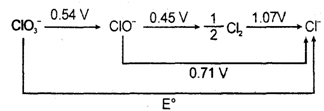The E° in the given diagram is,

Solution: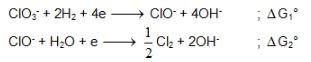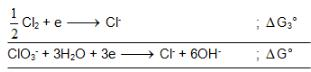∴   ΔG0= ΔG10 +ΔG20 + ΔG30

-6FE0. = -4F x 0.54 - 1F x 1.07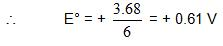QUESTION: 2

### What is cell entropy change of the following cell? Pt(s) | H2(g) | CH3COOH, HCl || KCl (aq) |Hg2Cl2| (s) | Hg P = 1 atm        0.1M                0.1M Emf of the cell is found to be 0.045 V at 298 K and temperature coefficient is  3.4 x10–4  VK–1 Given Ka (CH3COOH) = 10–5 M

Solution: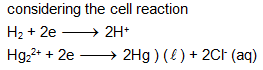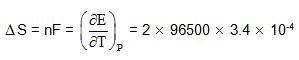= 65.223J/K/mole

QUESTION: 3

### Following cell has EMF 0.7995 V. Pt | H2 (1 atm) | HNO3 (1M) || AgNO3 (1M) | Ag If we add enough KCl to the Ag cell so that the final Cl- is 1M. Now the measured emf of the cell is 0.222 V. The Ksp of AgCl would be :

Solution: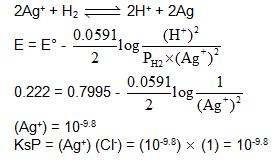QUESTION: 4

The solubility of [Co(NH3)4Cl2] CIO4_________ if the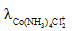= 50,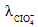= 70, and the measured resistance was 33.5Ω in a cell with cell constant of 0.20 is ____.

Solution:

The correct answer is option B
Given,
λCo(NH3)4Cl2+=50       λClo-4 =70
λ∞= λCo(NH3)4Cl2+  + λClo-4
λ∞= 50             + 70
λ∞=120
(x) Cell constant = 1/A
0.02 = l/A
Resistance(R)   =33.5Ω
K =c.x              (x = is cell constant)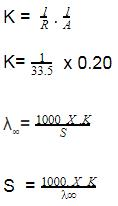S  =49.7 mol/L

QUESTION: 5

We have taken a saturated solution of AgBr.Ksp of AgBr is 12 x 10 – 14 . If 10 – 7 mole of AgNO3 are added to 1 litre of this solution then the conductivity of this solution in terms of  10 – 7 Sm – 1 units will be

[given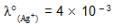Sm2 mol-1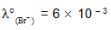Smmol-1, 5 x 10-3 Sm2mol-1]

Solution:

The solubility of Agbr in presence of 10-7 molar AgNo3 is 3 x 10-7 M. therefore [Br] 3 x 10-4 M3, [Ag+] = 4 x 10-4 m3 and [No3-]= 10-4 m3

Therefore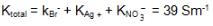QUESTION: 6

At 298K the standard free energy of formation of H2O(L) is – 237.20kJ/mole while that of its ionisation into H+ ion and hydroxyl ions is 80 kJ/mole, then the emf of the following cell at 298 K will be

H2(g,1 bar) | H+ (1M) || OH (1M) | O2 (g, 1bar)

Solution:

Cell reaction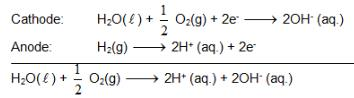also we have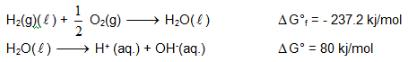Hence for cell reaction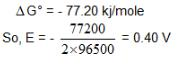QUESTION: 7

Which of the following cell can produce more electric work.

Solution: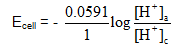For Ecell to be highest [H+]a should be lower and [H+]c should be higher and that why anode compartment should be more basic and cathodic compartment should be acidic.

QUESTION: 8

A hydrogen electrodes is immersed in a solution with pH = 0 (HCl). By how much will the potential (reduction) change if an equivalent amount of NaOH is added to the solution. (Take PH2 = 1 atm) T = 298 K.

Solution:

pH changes from 0 to 7

∴ [H+] changes from 1 to 10-7 M

Accordingly Ered. Decrease by 0.059 log 10-7 i.e 0.059 x (-7) = -0.41volt

QUESTION: 9

At what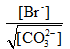does the following cell have its reaction at equilibrium?

Ag(s) | Ag2CO3(s) | Na2CO3 (aq) || KBr(aq) | AgBr(s) | Ag(s)

KSP =  8 x 10 – 12 for  Ag2CO3 and KSP = 4 x 10 – 13 for AgBr

Solution: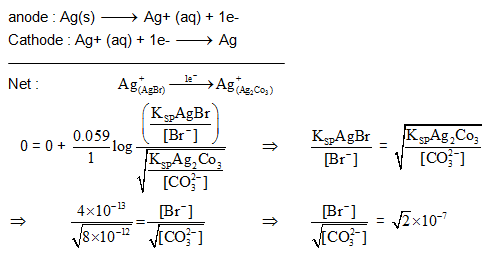QUESTION: 10

Calculate the EMF of the cell at 298 K

Pt|H2(1atm)|NaOH(xM),NaCl(xM)|AgCl(s)|Ag

If E°cl-/AgCl/Ag = + 0.222 V

Solution: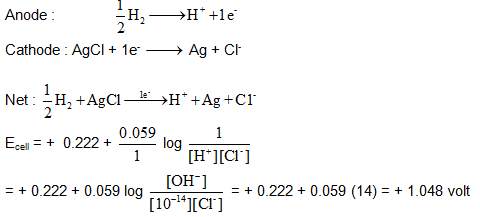QUESTION: 11

A current of 0.1A was passed for 2hr through a solution cuprocyanide and 0.3745g f copper was deposited on the cathode. Calculate the current efficiency for the copper deposition.

Solution: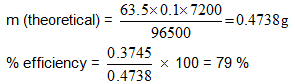QUESTION: 12

With t taken in seconds and I taken in Amp, the variation of I follows the equation

t2 + I2 = 25

what amount of Ag will be electrodeposited with this current flowing in the interval 0-5 second ? (Ag : 108)

Solution: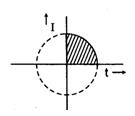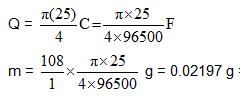=21.97mg = 22mg

QUESTION: 13

A resistance of 50Ω is registered when two electrodes are suspended into a beaker containing a dilute solution of a strong electrolyte such that exactly half of the them are submerged into solution. If the solution is diluted by adding pure water (negligible conductivity) so as to just completely submerge the electrodes, the new resistance offered by the solution would be

Solution: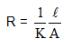the k is halved while the A is doubled. Hence R remain 50 Ω.

QUESTION: 14

The standard reduction potential of a silver chloride electrode is 0.2 V and that of a silver electrode is 0.79 V. The maximum amount of AgCl that can dissolve in 106 L of a 0.1 M AgNO3 solution is

Solution: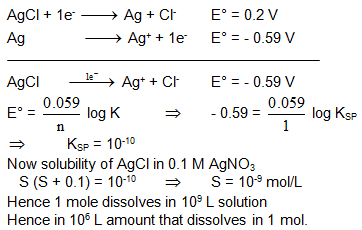QUESTION: 15

Calculate the cell EMF in mV for

Pt|H2(1atm) |HCl(0.01M)|AgCl(s)| Ag(s)        at 298 K

If ΔG°r values are at 25°C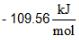for AgCl(s) and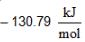for H+ + Cl-) (aq)

Solution: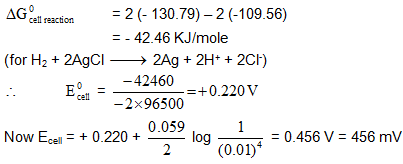QUESTION: 16

Adiponitrile is manufactured electrolytically from acrylonitrile

CH2 = CHCN → CN – (CH2)4 – CN

How many kg of adiponitrile (molecular mass = 108) is produced in 9.65 hr using a current of 3750 A with 80% efficiency

Solution: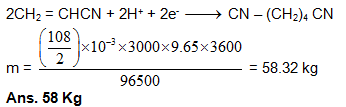QUESTION: 17

It is observed that the voltage of a galvanic cell using the reaction M(s) + xH+→ Mx+ + X/2H2 varies linearly with the log of the square root of the hydrogen pressure and the cube root of the Mx+ concentration. The value of x is

Solution: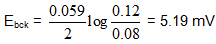QUESTION: 18

Acetic acid has Ka = 1.8 X 10 – 5 while formic acid had Ka = 2.1 X 10–4. What would be the magnitude of the emf of the cell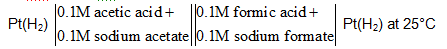Solution: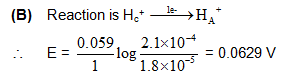QUESTION: 19

Consider the cell Ag(s)|AgBr(s)|Br-(aq)||AgCl(s)|Cl-(aq)|Ag(s) at 25°C. The solubility product constants of AgBr & AgCl are respectively 5 X 10 – 13 & 1 X 10 – 10. For what ratio of the concentrations of Br- & Cl- ions would the emf of the cell be zero ?

Solution: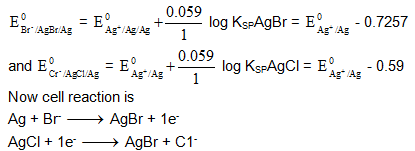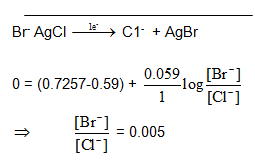QUESTION: 20

Value of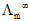for SrCl2 in water at 25°C from the following data: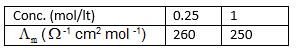Solution: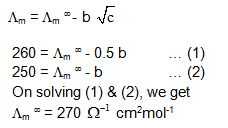QUESTION: 21

Calculate the useful work of the reaction Ag(s) + 1/2Cl2(g) → AgCl(s)
Given  E°cl2/cl- = + 1.36 V,       E°AgCl/Ag,Cl- = 0.22 V

If         Pcl2 = 1 atm     and T = 298 K

Solution:

(1) AgCl (s) + e → Ag(s) + Cl-         E0 = 22 V

(2) 1/2 Cl2 + e → Cl-             E= 1.36V

we get

Ag(s) + 1/2Cl2 (g) →AgCl(s)          E0cell = 1.14V

QUESTION: 22

Which of these ions Cu+, Co3+, Fe2+ is stable in aqueous medium.

Given :

Cu2+/Cu+ = 0.15 volt ;            E°Cu+/Cu = 0.53 V ;       E°Co3+/Co2+ = 1.82 V ;

Fe3+/Fe2+ = 0.77 V ;   E°Fe2+Fe = - 0.44 V ;    E°O2,H+/H2O = 1.23 V

Solution:

if reduction potential of metal ion is greater then O2/H2O couple, the ion is stable in water. So Co3+ is stable in water

QUESTION: 23

Select the correct statement if -

Mg2+/Mg = - 2.4V,      E°Sn4+/Sn2+ = 0.1 V,     E°MnO4-,H+/Mn2+ = 1.5 V, E° I2/I- = 0.5 V    Here,

Solution:

[Hint: Reverse of (B) & (C) is spontaneous; weakest Oxidizing Agent here is Mg2+]

QUESTION: 24

What is the value of pKb (CH3COOH-) if λm = 390 & λm = 7.8 for 0.04 of a CH3COOH at 25°C

Solution: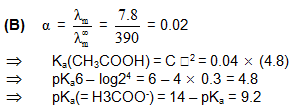QUESTION: 25

The temperature coefficient of a standard Cd-cell is – 5.0X10– 5 Vk– 1 whose emf at 25°C is 1.018 V. During the cell operation, the temperature will -

Solution: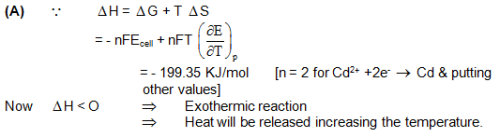QUESTION: 26

A cell Ag | Ag+ || Cu++ | Cu initially contains 2M Ag+ and 2M Cu++ ions. The charger in cell potential after the passage of 10 amp current for 4825 sec is:

Solution: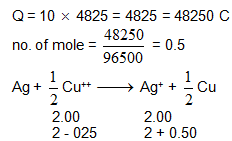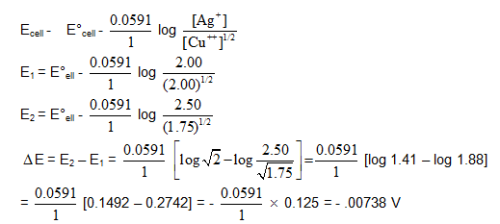QUESTION: 27

For the cell (at 298 K)

Ag(s) | AgCl(s) | Cl-(aq) || AgNO3(aq) | Ag(s)

Which of the following is correct –

Solution:

Conductivity is high due to [H+]

QUESTION: 28

During an electrolysis of conc. H2SO4, perdissulphuric acid (H2S2O8) and O2 from in equimolar amount. The amount of H2 that will form simultaneously will be (2H2SO4 → H2S2O8 + 2H+ + 2e-)

Solution: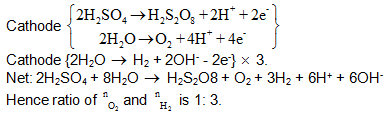QUESTION: 29

Statement-1: In electrochemical cell, we cannot use KCI in the salt bridge if anodic  or cathodic compartment consists of Ag+ of Pb2+ ion.

Statement-2: Salt bridge is employed to maintain the electrical neutrality and to minimize the liquid-liquid junction potential.

Solution:

Both (S – 1) & (S – 2) are true and (S – 2) is not the correct explanation of    (S – 1)

QUESTION: 30

Statement-1: Zinc protect the iron better than tin even after it cracks.

Statement-2:OPzn < E°OPfe But E°OPSn > E°OPfe

Solution: